Next: 3.1.2 The Drift-Diffusion Transport Up: 3.1 Sets of Partial Previous: 3.1 Sets of Partial

## 3.1.1 The Basic Semiconductor Equations

The basic equations solved in a device simulator are the Poisson equation and the continuity equations for electrons and holes.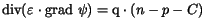(3.1)(3.2)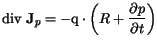(3.3)

The unknown quantities of this equation system are the electrostatic potential,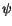, and the electron and hole concentrations,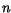and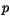, respectively.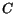denotes the net concentration of the ionized dopants and other charged defects,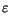is the dielectric permittivity of the semiconductor, and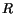is the net recombination rate.

Vassil Palankovski
2001-02-28